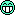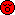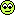programming forums Java Mobile Certification Databases Caching Books Engineering Micro Controllers OS Languages Paradigms IDEs Build Tools Frameworks Application Servers Open Source This Site Careers Other Pie Elite all forums
this forum made possible by our volunteer staff, including ...
Marshals:
• Campbell Ritchie
• Paul Clapham
• Ron McLeod
• Jeanne Boyarsky
• Tim Cooke
Sheriffs:
• Liutauras Vilda
• paul wheaton
• Henry Wong
Saloon Keepers:
• Tim Moores
• Tim Holloway
• Stephan van Hulst
• Carey Brown
• Frits Walraven
Bartenders:
• Piet Souris
• Himai Minh

# Math.random

Ranch Hand
Posts: 35
••Number of slices to send:
Optional 'thank-you' note:
••Thanx for your reply i learned quite alot but the formula u told is like for a range of 10 numbers from 30-40:
Math.random()*10+30
but it is not working in that way.
only the formula i mentioned before is working properly and i don't know what is the logic behind that formula

[This message has been edited by Argm Mastoi (edited October 18, 2001).]

Ranch Hand
Posts: 515
••Number of slices to send:
Optional 'thank-you' note:
••It works like this.. the Math.random() method creates a number between 0 and 1. so it will randomly create a floating point number. You then can create any number from that by adjusting the range and starting point. The range is what you multiply by and the starting point is what you add on after this. Example
I want to create a number from 1 to 10
I would do the following
Math.random()*10
so I get a .31 for my Math.random part. I do the multiply and get 3.1
So my number is now 3.
Next I decide... no infact I need a number from 30 to 40. My range is 10 and my starting point is 30. So I then start again
Lets say again I get .31
I multiply by 10 and get 3.1 .. which infact is cut off to be 3
then I add my base of 30.
I get 33.
Get it!?
So in your case you have the following
---------------------------------
Math.random()*(1000-500)+500
the first part creates a number between 0 and 1
lets again say .31
The 1000-500 is calculated first to get 500 so we know your range is 500. We take your number and multiply it by 500. .31 * 500 is 155. Then we add 500 to it and get 655. It works great. So your formulate says this...
Math.random()*(endingRangeNumber-StartingRangeNumber)+StartingPoint
Or if you want to simplify it
Math.random()*(rangeOfNumbersInYourSet) + startingPoint
So if you wanted a number between 300 and 350, you would have this
(Math.random() * 50) + 300
Hope this helps.

Dale

------------------
By failing to prepare, you are preparing to fail.
Benjamin Franklin (1706 - 1790)
[This message has been edited by Dale DeMott (edited October 18, 2001).]

Argm Mastoi
Ranch Hand
Posts: 35
••Number of slices to send:
Optional 'thank-you' note:
••thanx alot, it was really a problem that u solved. i understood the thing quite properly,
Argm.

Greenhorn
Posts: 20
••Number of slices to send:
Optional 'thank-you' note:
••One thing was overlooked with the formula... it only gives numbers ranging from 30-39 and not till 40. Does anyone know? Thanks in advance.

Margaret Tan
Greenhorn
Posts: 20
••Number of slices to send:
Optional 'thank-you' note:
••I have to add that it only range till 39 since the Math.random() method returns a DOUBLE value greater than or EQUAL to 0.0 but LESS THAN 1.0 (not the less than, there's no equal to)

Ranch Hand
Posts: 158
••Number of slices to send:
Optional 'thank-you' note:
••You are correct Margaret, the Math.random () function returns a double value greater than or equal to 0.0 and strictly less than 1.0. and if you multiply that number by a range number, you'll get random numbers less than the range max, but never exactly the range max. Now if you cast the random numbers to ints like so:
(int) (Math.random () * rangeMax);
then you'll get a list of integers between 0 and rangeMax. If you want to include the rangeMax, you'll have to add one to it, then multiply by Math.random ().------------------
Michael J Bruesch
Codito, ergo sum...
I code, therefore I am.
http://www.geocities.com/mjbruesch

Margaret Tan
Greenhorn
Posts: 20
••Number of slices to send:
Optional 'thank-you' note:
••Thanks for supporting my answer Michael.. but I don't understand how your formula can give the range from 30-40?
(int)(Math.random()*10 + 30)
this formula would give answers from 30-39 but how do you modify this to make it generate numbers from 30-40?
Thanks..

Margaret Tan
Greenhorn
Posts: 20
••Number of slices to send:
Optional 'thank-you' note:
••Sorry Mike,
Ok, can you give me a formula where you can generate numbers from rangeMin to rangeMax? ThanksMichael Bruesch
Ranch Hand
Posts: 158
••Number of slices to send:
Optional 'thank-you' note:
••if you need the numbers in your range to be integers, then the following should suffice:------------------
Michael J Bruesch
Codito, ergo sum...
I code, therefore I am.
http://www.geocities.com/mjbruesch

Margaret Tan
Greenhorn
Posts: 20
••Number of slices to send:
Optional 'thank-you' note:
••uh uh , doesn't work Mike, here is the code and the output
class TestRandom{
public static void main(String args[]){
int num, i=0;
int rangeMin=30;
int rangeMax=40;
do{
//generates random number starting from rangeMin to rangeMax
num = (int)(Math.random()*(rangeMax+1)+rangeMin);
System.out.println(num);
}while(num!=40);
}
}
output from DOS prompt.. see how it generate a number more than 40?
35
48
59
53
48
33
40

Michael Bruesch
Ranch Hand
Posts: 158
••Number of slices to send:
Optional 'thank-you' note:
••Whooops, sorry Margaret, had the close parenthesis in the wrong place, should be:

Sorry!------------------
Michael J Bruesch
Codito, ergo sum...
I code, therefore I am.
http://www.geocities.com/mjbruesch

Michael Bruesch
Ranch Hand
Posts: 158
••Number of slices to send:
Optional 'thank-you' note:
••Alright, I'm all confused. I'm sorry, the variable name shouldn't be rangeMax, it should be just range. In your case, the range of numbers is 10, ie. from 30 to 40. So substitue range for rangeMax and you're homefree. I compiled and ran this code to make sure it's correct:

------------------
Michael J Bruesch
Codito, ergo sum...
I code, therefore I am.
http://www.geocities.com/mjbruesch

Michael Bruesch
Ranch Hand
Posts: 158
••Number of slices to send:
Optional 'thank-you' note:
••And actually, if you think about it, from 30 to 40 is actually 11 numbers possible, so your range is actually 11 and not 10.

Argm Mastoi
Ranch Hand
Posts: 35
••Number of slices to send:
Optional 'thank-you' note:
••hi Margaret,
If you've closely looked over the code, provided by Dale, you can easily understand that in the formula, there's an starting point(where to start?) and inspite of the ending point the range of number is given(means upto what number of numbers it has to generate) so its you to tell the range whether 10 or 11.
for numbers 30-40:
(int)((Math.random()*11)+30)
I hope this formula will work out.
Argm

Michael Bruesch
Ranch Hand
Posts: 158
••Number of slices to send:
Optional 'thank-you' note:
••That's what I was trying to say, only it took about 4 posts to do it.But they say, it's not the destination, it's the journey....or something like that.
Happy random number finding!

SheriffPosts: 16936
286••Number of slices to send:
Optional 'thank-you' note:
••Don't know if any changes have been made to Math.random() recently but I have read advice from one author to use the java.util.Random class instead of Math.random() as the latter has a flaw in its algorithm.

------------------
Junilu Lacar
Sun Certified Programmer for the Java� 2 PlatformDale DeMott
Ranch Hand
Posts: 515
••Number of slices to send:
Optional 'thank-you' note:
••At the risk of being redundant I wanted to clarify what I was saying so you could understand it a bit more. Again the formula is this
Math.random()*(rangeOfNumbersInYourSet) + startingPoint
If you wanted to create a list of numbers from 30 to 40 (including the ending points 30 and 40), there are 11 numbers in the list so your range would have 11 in it. You have to remember when you are subtracting numbers, you are actually counting the spaces between the numbers. Not the numbers themselves. If you want to count the numbers, you always need to add 1. Example - If I want a range of numbers from 0 to 4 (including 4), I need to tell the system 5. Reason being is that there are 5 numbers in my set. 0, 1, 2, 3, 4. I should have altered my formula to state the following
Math.random()*(rangeOfNumbersInYourSet + 1) + startingPoint
This usually appropriate since most people assume the range will include the last element in the list. So again if I wanted a range of numbers from 300 to 350, (including 350) it would look like this.
Math.random()*(350 - 300 + 1) + 300
Regards,
Dale DeMott

------------------
By failing to prepare, you are preparing to fail.
Benjamin Franklin (1706 - 1790)Whip out those weird instruments of science and probe away! I think it's a tiny ad: free, earth-friendly heat - a kickstarter for putting coin in your pocket while saving the earth https://coderanch.com/t/751654/free-earth-friendly-heat-kickstarter
••